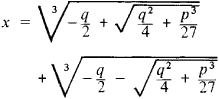# Cardano's Formula

Also found in: Dictionary.
The following article is from The Great Soviet Encyclopedia (1979). It might be outdated or ideologically biased.

## Cardano’s Formula

a formula for finding the roots of the reduced cubic equation

x3 + px + q= 0

The formula has the following form:Any cube root has three values, among which only one is real. The values of the cube roots in Cardano’s formula should be taken such that their product be equal to —p/3; it is precisely these values that must be summed to obtain the root of the equation. In this way it is possible to find the three roots of the equation. Cardano’s formula is named for G. Cardano and was first published by him in 1545, although the question of whether it was found by Cardano himself or copied by him from N. Tar-taglia, or discovered even earlier (c. 1515) by S. dal Ferro, is impossible to answer with complete certainty.